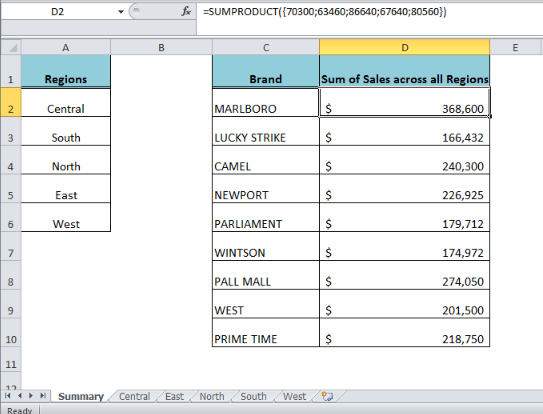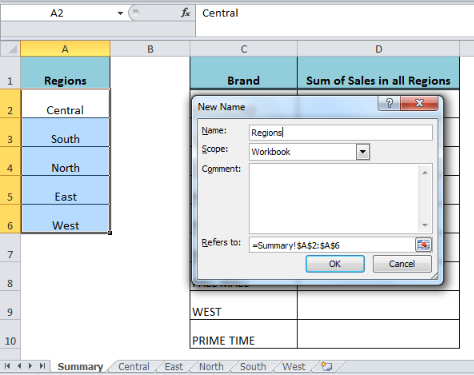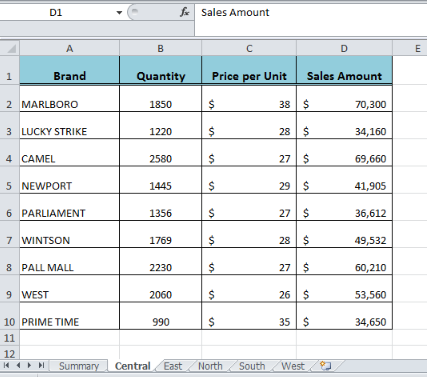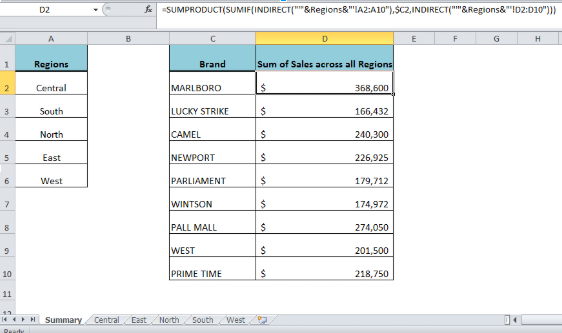Get instant live expert help with Excel or Google Sheets“My Excelchat expert helped me in less than 20 minutes, saving me what would have been 5 hours of work!”

#### Post your problem and you’ll get Expert help in seconds.

Your message must be at least 40 characters
Our professional Expert are available now. Your privacy is guaranteed.

# How to Use the SUMIF Function Across Multiple Sheets

When the data is spread out in different worksheets in similar ranges of cells, we can add categorize the data by using the SUMIF function across multiple sheets. This can be done by nesting the SUMIF function and the INDIRECT function. This step by step tutorial will assist all levels of Excel users in summarizing data across multiple sheets.Figure 1. Final result

## Formula Syntax

The generic formula syntax is as follows:

`=SUMPRODUCT(SUMIF(INDIRECT("'"&named_range&"'!range"),criteria,INDIRECT("'"&named_range&"'!sum_range")))`

Where;

• Named_range: It is the named range of all the different worksheets across which we want to conditionally sum the values
• Range: It is a range of identical cells across multiple sheets on which we want to test the criteria
• Sum_range: It is a range of identical cells across multiple sheets from where we want to sum the values

## Defining Named Range

We will list out all the worksheets in a range of cells and then we will define a named range. In our example, we have sales dataset of various cigarettes brands in different regions where each region’s sales data is recorded across multiple worksheets. We will prepare a list of all regions’ worksheets names on summary worksheets and define their named range as Regions as follows:

• Inset worksheets names in a range of cells, such as A2:A6 in this example
• Select this range of cells
• Go to Formula tab > Select Define Name in Defined Names section
• A New Name dialog box appears. Inset name of this range, such as Regions in our example
• Click OKFigure 2. Defining named range of worksheets

## Identical Range of cells

On each region’s worksheet, the sales data of various cigarettes brands should be in the identical range of cells, as it looks like this:Figure 3. Sales data in identical cells across multiple sheets

## Applying the SUMIF Function Across Multiple Sheets

On the Summary worksheet, in cell D2 we will apply the following formula and copy down to other values of brands names to summarise their sales data across all the regions:

`=SUMPRODUCT(SUMIF(INDIRECT("'"&Regions&"'!A2:A10"),\$C2,INDIRECT("'"&Regions&"'!D2:D10")))`Figure 4. Applying the SUMIF formula across multiple sheets

## How this Formula Works

We use the named range of multiple sheets to create a reference for each sheet and merge these sheets’ names with identical ranges across these multiple sheets. The INDIRECT function is used to identify these sheets names and identical ranges correctly. As the named range “Regions” contains various values. The SUMIF function returns the results in an array of summed values for each brand (criteria value) across multiple sheets. Finally, the SUMPRODUCT function sums all the values of this resulting array returned by the SUMIF function for each criteria value across multiple sheets. As there are five regions in our example, so there might be five resulting values for each brand, that looks like this:

`=SUMPRODUCT({70300;63460;86640;67640;80560})`Figure 5. The resulting array of values returned by the SUMIF function

## Instant Connection to an Expert through our Excelchat Service:

Most of the time, the problem you will need to solve will be more complex than a simple application of a formula or function. If you want to save hours of research and frustration, try our live Excelchat service! Our Excel Experts are available 24/7 to answer any Excel question you may have. We guarantee a connection within 30 seconds and a customized solution within 20 minutes.

### Did this post not answer your question? Get a solution from connecting with the expert.Another blog reader asked this question today on Excelchat:
Solution examplesamending a formula so that there is another criteria and if it does not meet this criteria then we stick with the original criteria
Solved by A. B. in 40 minsI need Column D to = Column C - Column A only if Column B has a 'Date' entered.
Solved by I. B. in 11 minsHello, I need help trying to create a sum of numerical values on a spreadsheet in which the only values that apply to the sum are when the status of another cell is a certain value (e.g. value is not in sum when status: closed, value is in sum when status: open)
Solved by T. W. in 18 minsI have a google sheet that takes information from a google form, that creates a row every time a form is submitted. Since it is a new row that is created, rather than filling in the next row down, the formulas I have applied to some of the columns do not work. The same is true when I try and link the data to another sheet to bypass this problem. This is probably quite a general functionality issue so I won't send the actual document but let me know if you need it
Solved by F. F. in 13 minsi have a table with Dates, First names , Last names and i want to count how many times a name occurs, but if a name occurs more than once on a particular date i need to only count it once. this is on excel and not google sheets
Solved by F. H. in 31 mins## Subscribe to Excelchat.coAnother blog reader asked this question today on Excelchat: